Next: References Up: Computer Vision IT412 Previous: Identifying connected components

Subsections

# Mathematical Morphology

Mathematical Morphology is a tool for extracting image components that are useful for representation and description. The technique was originally developed by Matheron and Serra  at the Ecole des Mines in Paris. It is a set-theoretic method of image analysis providing a quantitative description of geometrical structures. (At the Ecole des Mines they were interested in analysing geological data and the structure of materials). Morphology can provide boundaries of objects, their skeletons, and their convex hulls. It is also useful for many pre- and post-processing techniques, especially in edge thinning and pruning.

Generally speaking most morphological operations are based on simple expanding and shrinking operations. The primary application of morphology occurs in binary images, though it is also used on grey level images. It can also be useful on range images. (A range image is one where grey levels represent the distance from the sensor to the objects in the scene rather than the intensity of light reflected from them).

## Set operations

The two basic morphological set transformations are erosion and dilation

These transformations involve the interaction between an image A (the object of interest) and a structuring set B, called the structuring element.

Typically the structuring element B is a circular disc in the plane, but it can be any shape. The image and structuring element sets need not be restricted to sets in the 2D plane, but could be defined in 1, 2, 3 (or higher) dimensions.

Let A and B be subsets of Z2. The translation of A by x is denoted Ax and is defined as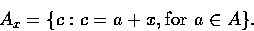The reflection of B, denoted, is defined as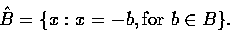The complement of A is denoted Ac, and the difference of two sets A and B is denoted A - B.

### Dilation

Dilation of the object A by the structuring element B is given by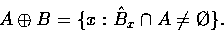The result is a new set made up of all points generated by obtaining the reflection of B about its origin and then shifting this relection by x.

Consider the example where A is a rectangle and B is a disc centred on the origin. (Note that if B is not centred on the origin we will get a translation of the object as well.) Since B is symmetric,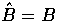.This definition becomes very intuitive when the structuring element B is viewed as a convolution mask.

### Erosion

Erosion of the object A by a structuring element B is given by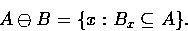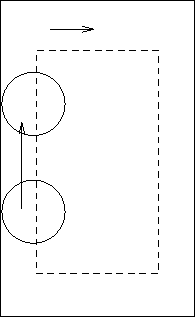Dilation and erosion are duals of each other with respect to set complementation and reflection. That is,To see this, consider first the left hand side: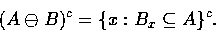Now, if Bx is contained in A, then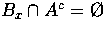, and so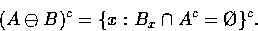But the complement of the set of all xs that satisfyis just the set of all xs such that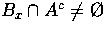. Thus### Applications of morphological operations

Erosion and dilation can be used in a variety of ways, in parallel and series, to give other transformations including thickening, thinning, skeletonisation and many others.

Two very important transformations are opening and closing. Now intuitively, dilation expands an image object and erosion shrinks it. Opening generally smooths a contour in an image, breaking narrow isthmuses and eliminating thin protrusions. Closing tends to narrow smooth sections of contours, fusing narrow breaks and long thin gulfs, eliminating small holes, and filling gaps in contours.

The opening of A by B, denoted by, is given by the erosion by B, followed by the dilation by B, that is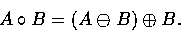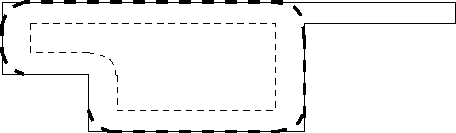Opening is like rounding from the inside': the opening of A by B is obtained by taking the union of all translates of B that fit inside A. Parts of A that are smaller than B are removed. Thus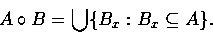Closing is the dual operation of opening and is denoted by. It is produced by the dilation of A by B, followed by the erosion by B: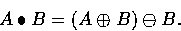This is like smoothing from the outside'. Holes are filled in and narrow valleys are closed'.

Just as with dilation and erosion, opening and closing are dual operations. That isThe opening operation satisfies the following properties:
1.is a subset of A.
2.
If C is a subset of D, then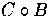is a subset of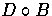.
3..
Similarly
1.
A is a subset of.
2.
If C is a subset of D, then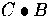is a subset of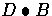.
3.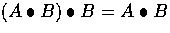.
Property 3, in both cases, is known as idempotency. It means that any application of the operation more than once will have no further effect on the result.

The morphological filter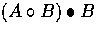can be used to eliminate salt and pepper' noise. Salt and pepper noise is random, uniformly distributed small noisy elements often found corrupting real images. It will appear as black dots or small blobs on a white background, and white dots or small blobs on the black object. The background noise is eliminated at the erosion stage, under the assumption that all noise components are physically smaller than the structuring element B. Erosion on its own will increase the size of the noise components on the object. However, these are eliminated at the closing operation.

The important thing to note is that morphological operations preserve the main geometric structures of the object. Only features smaller than' the structuring element are affected by transformations. All other features at larger scales' are not degraded. (This is not the case with linear transformations, such as convolution).

The boundary of a set A, denoted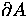, can be obtained by first eroding A with B, where B is a suitable structuring element, and then performing the set difference between A and its erosion. That is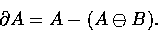Typically, B would be a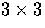matrix of 1s.

Region filling can be accomplished iteratively using dilations, complementation, and intersections. Suppose we have an image A containing a subset whose elements are 8-connected boundary points of a region. Beginning with a point p inside the boundary, the objective is to fill the entire region with 1s.

Since, by assumption, all non-boundary points are labeled 0, we begin by assigning 1 to p, and then constructwhere X0 = p, and B is the `cross' structuring element shown in figure 8. The algorithm terminates when Xk = Xk-1. The set union of Xk and A contains the filled set and its boundary.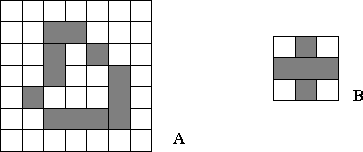Likewise, connected components can also be extracted using morphological operations. If Y represents a connected component in an image A and a point p in Y is known, then the following iterative expression yields all the elements of Y: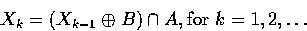where X0 = p and B is amatrix of 1s. If Xk = Xk-1 the algorithm has converged and we let Y = Xk.

An important step in representing the structural shape of a planar region is to reduce it to a graph. This is very commonly used in robot path planning. This reduction is most commonly achieved by reducing the region to its skeleton.

The skeleton of a region is defined by the medial axis transformation (MAT). The MAT of a region R with border B is defined as follows: for each point p in R, we find its closest neighbour in B. If p has more than one such closest neighbour, then p belongs to the medial axis (or skeleton) of R. Of course, closest depends on the metric used. Figure 9 shows some examples with the usual Euclidean metric.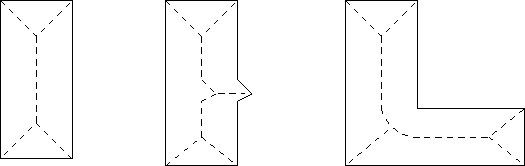Direct implementation of the MAT is computationally prohibitive. However, the skeleton of a set can be expressed in terms of erosions and openings. Thus, it can be shown that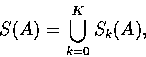where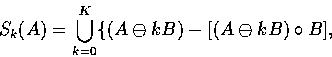B is a structuring element,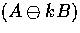indicates k successive erosions of A, and K is the last iterative step before A erodes to an empty set.

Thus A can be reconstructed from its skeleton subsets Sk(A) using the equation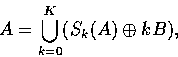where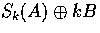represents k successive dilations of Sk(A).Next: References Up: Computer Vision IT412 Previous: Identifying connected components
Robyn Owens
10/29/1997